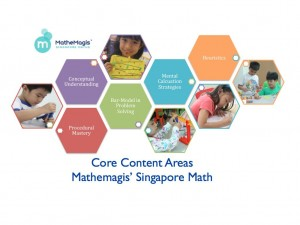﻿ MatheMagis Singapore Maths » Blog Archive » Top 5 reasons why your child should learn Singapore Math

### Top 5 reasons why your child should learn Singapore MathMathemagis’ Singapore Math covers core content areas of Math learning and skills development.

### 1. Conceptual Understanding

Students learn the whys before the hows and understand the underlying mathematical principles behind common shortcuts and formulas.  An emphasis on conceptual understanding allows students to solve seemingly complex problems with ease.
Traditional Math:  5 x 3 = ?
Singapore Math:  5 x 3 = 5 x 2 + ?
Level 2 (grade 2) students will be able to answer this based on a conceptual understanding of multiplication (no algebra, please).

### 2. Procedural Mastery

Knowledge and mastery of mathematical procedures are developed AFTER conceptual understanding.

### 3. Mental Computations strategy

Students learn various mental computation strategies on top of pen and paper calculations.

### 4. Use of Bar model in word problems.

Students  develop visualization and analytical skills needed to learn higher mathematics such as trigonometry and calculus through the use of bar-models as early as level 1 (grade 1).
Traditional Math Algebra Word Problem : Jose’s present age is 1/7 of his father’s age.  His father will be 61 years old in 5 years’ time. In how many years’ time will Jose’s age be 1/5 of his father’s age?
Singapore Math Level 5 (Grade 5) Word Problem: Jose’s present age is 1/7 of his father’s age.  His father will be 61 years old in 5 years’ time. In how many years’ time will Jose’s age be 1/5 of his father’s age?
Level 5 students will be able to answer the above problem using the bar-model approach.  Yes, without using algebra!  Singapore math focuses on visualization, analysis and strategies for breaking down complex word problems — allowing grade 5 students to answer questions normally reserved for Algebra.

### 5. Heuristics

Mathemagis teaches various strategies that can be applied to word problems aside from the bar-model approach.  This makes students more versatile and exposed to a wide range of word problems both mathematical and practical.
The Mathemagis Singapore math program covers all content areas and skills development in mastering primary school math and preparing students for higher order math such as Algebra, Trigonometry and Calculus.